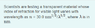# Thin film intereference

• AlexKappa

## Homework Statement

[/B]
Scientists are testing a transparent material whose index of refraction for visible light varies with wavelength as n = 30.0 nm^(1/2)/λ^(1/2), where λ is in nm.

A 310-nm-thick coating is placed on glass (n = 1.50) what visible wavelengths will the reflected light have maximum constructive interference?

## Homework Equations

λ_constructive = (2nd)/m where m = 1,2,3,4
Δφ = 2π*(2nd)/λ

d is thickness of film[/B]

## The Attempt at a Solution

[/B]
I am really confused by the index of refraction notation n = 30.0 nm^(1/2)/λ^(1/2), but what I got out was that in the visible spectrum, n < 1. So you have air where n = 1. the film where n < 1, and glass where n = 1.5 . So light will reflect off the film with no phase difference, and light reflects off the glass with a phase shift of π. So the light has to travel 2d and have a phase shift of mπ (m = 1,3,5...) to have constructive interference.

π = 2π*(2 * (sqrt(30nm)/(sqrt(λ))) * 310nm) / λ

using a calculator I got λ = 358.6nm

using 3π, I got λ = 191.4nm which isn't in the visible spectrum

This answer is sadly wrong because the online answer box says there are two wavelengths that will have constructive interference.

#### Attachments

•fafasfafas.png
3.4 KB · Views: 507